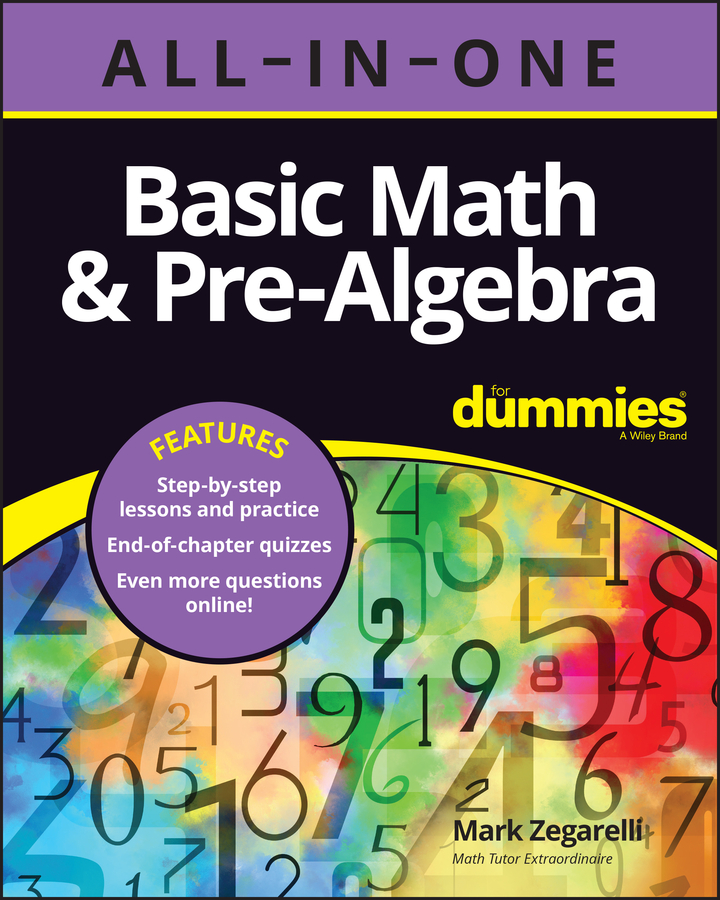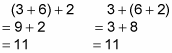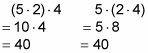##### Basic Math & Pre-Algebra All-in-One For Dummies (+ Chapter Quizzes Online)Addition and multiplication are both associative operations, which means that you can group them differently without changing the result. This property of addition and multiplication is also called the associative property. Here’s an example of how addition is associative. Suppose you want to add 3 + 6 + 2. You can solve this problem in two ways:In the first case, you start by adding 3 + 6 and then add 2. In the second case, you start by adding 6 + 2 and then add 3. Either way, the sum is 11.

And here’s an example of how multiplication is associative. Suppose you want to multiply 5 2 4. You can solve this problem in two ways:In the first case, you start by multiplying 5 2 and then multiply by 4. In the second case, you start by multiplying 2 4 and then multiply by 5. Either way, the product is 40. In contrast, subtraction and division are nonassociative operations. This means that grouping them in different ways changes the result.

Don’t confuse the commutative property with the associative property. The commutative property tells you that it’s okay to switch around two numbers that you’re adding or multiplying. The associative property tells you that it’s okay to regroup three numbers using parentheses.

Taken together, the commutative and associative properties allow you to completely rearrange and regroup a string of numbers that you’re adding or multiplying without changing the result.